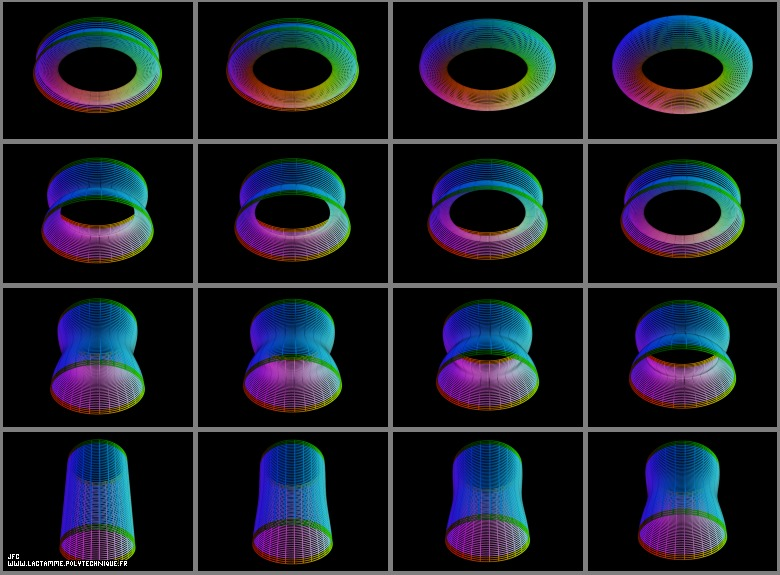Many surfaces -bidimensional manifolds- in a tridimensional space can be defined using a set of three equations:
```                    X = Fx(u,v)
```
```                    Y = Fy(u,v)
```
```                    Z = Fz(u,v)
```
with:
```                    u ∈ [Umin,Umax]
```
```                    v ∈ [Vmin,Vmax]
```
[Umin,Umax]*[Vmin,Vmax] then defined a bidimensional rectangular domain D.
```                       v ^
|
V    |...... ---------------------------
max |      |+++++++++++++++++++++++++++|
|      |+++++++++++++++++++++++++++|
|      |+++++++++++++++++++++++++++|
|      |+++++++++++++++++++++++++++|
|      |+++++++++++++++++++++++++++|
|      |+++++++++++++++++++++++++++|
|      |+++++++++++++++++++++++++++|
|      |+++++++++++++++++++++++++++|
|      |+++++++++++++++++++++++++++|
V    |...... ---------------------------
min |      :                           :
|      :                           :
O------------------------------------------------->
U                           U              u
min                         max
```

If D is sampled by means of a bidimensional rectangular grid (made of Nu*Nv points), the three {X,Y,Z} coordinates can be defined by means of three rectangular matrices:
```                    X = Mx(i,j)
```
```                    Y = My(i,j)
```
```                    Z = Mz(i,j)
```
with:
```                    i = f(u,Umin,Umax,Nu)
```
```                    j = g(v,Vmin,Vmax,Nv)
```
where 'f' and 'g' denote two obvious linear functions...

[Plus d'informations sur ce processus]

For the interpolation between a cylinder and a torus, the three sets of {X,Y,Z} fields/matrices are as follows:

See some of the interpolated surfaces: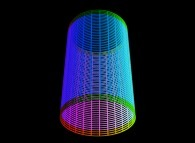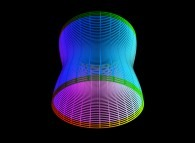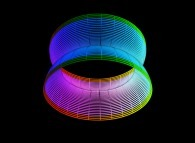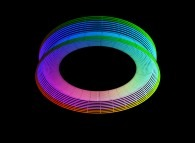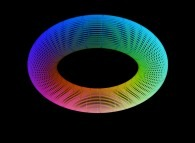(CMAP28 WWW site: this page was created on 11/13/2008 and last updated on 04/16/2023 20:52:10 -CEST-)

[More information about that kind of picture and/or process [Plus d'informations sur ce type d'image et/ou de processus]]

[See the generator of this picture [Voir le générateur de cette image]]

[See all related pictures (including this one) [Voir toutes les images associées (incluant celle-ci)]]

[Go back to AVirtualMachineForExploringSpaceTimeAndBeyond [Retour à AVirtualMachineForExploringSpaceTimeAndBeyond]]

[The Y2K Bug [Le bug de l'an 2000]]

[Site Map, Help and Search [Plan du Site, Aide et Recherche]]
[Mail [Courrier]]
[About Pictures and Animations [A Propos des Images et des Animations]]